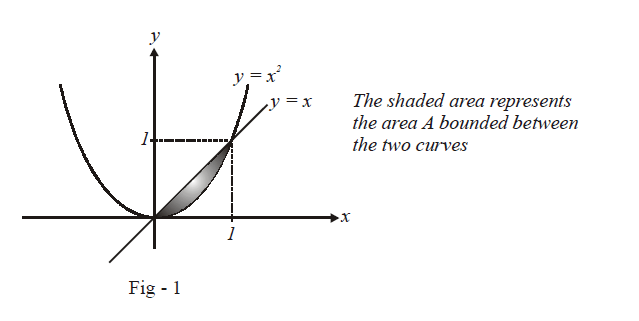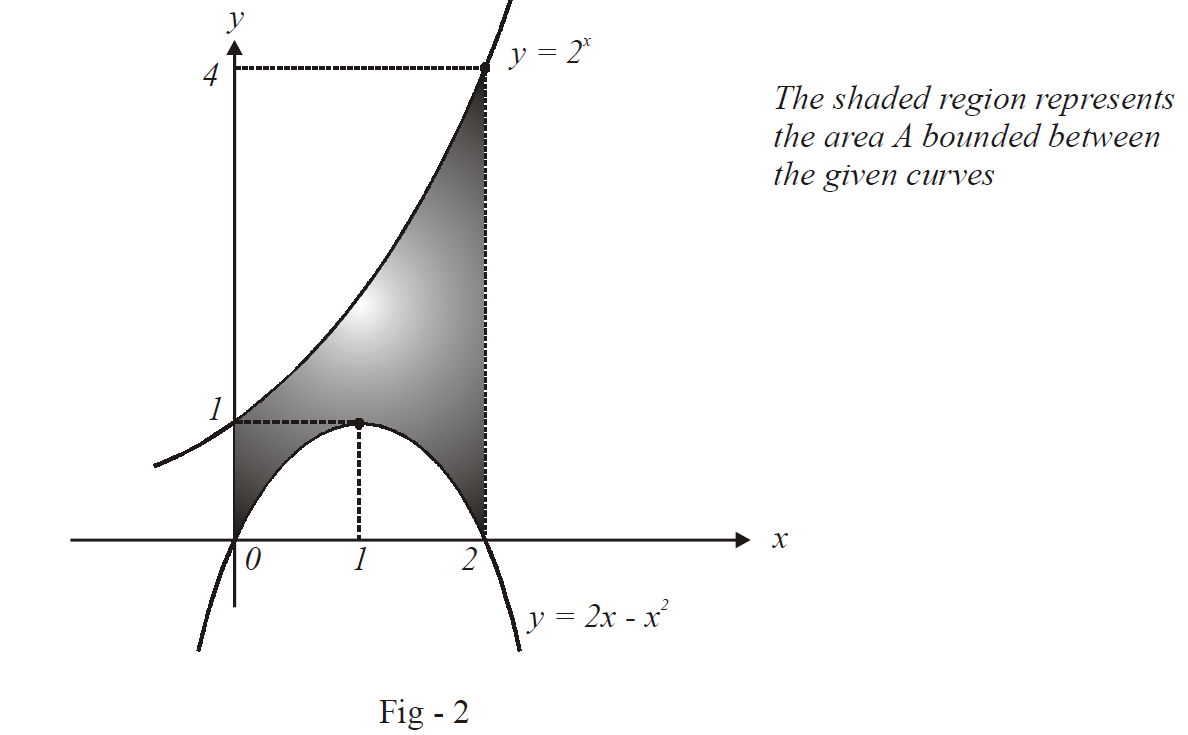# Problems On Areas Set-1

Go back to  'Areas and Volumes'

This is the chapter where we will actually put our knowledgeof (definite) integration to practical use. We will calculate areas undercurves, areas bounded by curves, volumes under surfaces, volumes bounded bysurfaces, etc. We will mostly be concerned with areas; however, we will delve alittle into calculating volumes for the sake of completeness.

Calculating areas and volumes is totally about calculatingdefinite integrals and involves no new concepts. You are advised to read thechapter on “Definite Integration” again if you are not very comfortablein evaluating integrals

Example – 1

Find the area bounded between the curves $$y{\rm{ }} = {\rm{ }}{x^2}\;{\rm{ and}}\;{\rm{ }}y{\rm{}} = {\rm{ }}x.$$

Solution: The bounded area is depicted in the figure below:Thus,

\begin{align}&A = \int\limits_0^1 {\left( {x -{x^2}} \right)} dx\\&\;\;\;\left. {\, = \left( {\frac{{{x^2}}}{2} -\frac{{{x^3}}}{3}} \right)} \right|_0^1\,\, = \,\frac{1}{{ 6}}sq\,\rm{units}\end{align}

Example – 2

Find the area of the region bounded between $$x{\rm{ }} = {\rm{ }}0,{\rm{ }}x{\rm{ }} = {\rm{}}2,{\rm{ }}y{\rm{ }} = {\rm{ }}{2^{x{\rm{ }}}}\;{\rm{ and}}\;y{\rm{ }} = {\rm{}}2x{\rm{ }}-{\rm{ }}{x^2}.$$

Solution: The bounded region has been sketched below.Verify for yourself the validity of the figure drawn:We have,

\begin{align}A &= \int\limits_0^2 {\left( {{2^x}- \left( {2x - {x^2}} \right)} \right)} dx\\& = \left. {\left({\frac{{{2^x}}}{{1n\,2}} - {x^2} + \frac{{{x^3}}}{3}} \right)} \right|_0^2\\&= \frac{4}{{1n\,2}} - 4 + \frac{8}{3} - \frac{1}{{1n\,2}}\\\\& = \left({\frac{3}{{1n}} - \frac{4}{3}} \right)sq.\,\rm{units}\,\end{align}